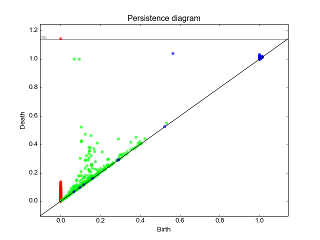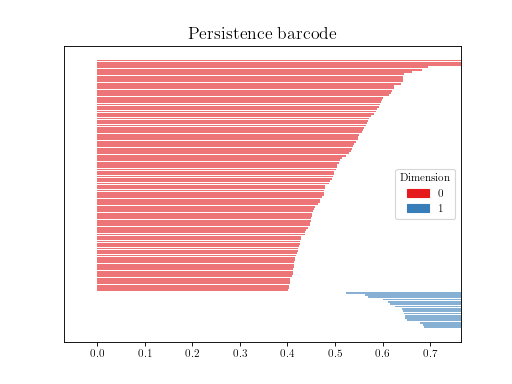Persistence graphical tools user manual¶

Definition¶These graphical tools comes on top of persistence results and allows the user to build easily persistence barcode, diagram or density.
Author: Vincent Rouvreau GUDHI 2.0.0 MIT matplotlib, numpy and scipy

Show persistence as a barcode¶

Note

this function requires matplotlib and numpy to be available

This function can display the persistence result as a barcode:

import gudhi

off_file = gudhi.__root_source_dir__ + '/data/points/tore3D_300.off'

rips_complex = gudhi.RipsComplex(points=point_cloud, max_edge_length=0.7)
simplex_tree = rips_complex.create_simplex_tree(max_dimension=3)
diag = simplex_tree.persistence(min_persistence=0.4)

plot = gudhi.plot_persistence_barcode(diag)
plot.show()Show persistence as a diagram¶

Note

this function requires matplotlib and numpy to be available

This function can display the persistence result as a diagram:

import gudhi

# rips_on_tore3D_1307.pers obtained from write_persistence_diagram method
persistence_file=gudhi.__root_source_dir__ + \
'/data/persistence_diagram/rips_on_tore3D_1307.pers'
plt = gudhi.plot_persistence_diagram(persistence_file=persistence_file,
legend=True)
plt.show()Persistence density¶

Note

this function requires matplotlib, numpy and scipy to be available

If you want more information on a specific dimension, for instance:

import gudhi

# rips_on_tore3D_1307.pers obtained from write_persistence_diagram method
persistence_file=gudhi.__root_source_dir__ + \
'/data/persistence_diagram/rips_on_tore3D_1307.pers'
plt = gudhi.plot_persistence_density(persistence_file=persistence_file,
max_intervals=0, dimension=1, legend=True)
plt.show()